Courses

# Test: Circles- 2

## 25 Questions MCQ Test Mathematics (Maths) Class 10 | Test: Circles- 2

Description
This mock test of Test: Circles- 2 for Class 10 helps you for every Class 10 entrance exam. This contains 25 Multiple Choice Questions for Class 10 Test: Circles- 2 (mcq) to study with solutions a complete question bank. The solved questions answers in this Test: Circles- 2 quiz give you a good mix of easy questions and tough questions. Class 10 students definitely take this Test: Circles- 2 exercise for a better result in the exam. You can find other Test: Circles- 2 extra questions, long questions & short questions for Class 10 on EduRev as well by searching above.
QUESTION: 1

### A point P is 10 cm from the centre of a circle. The length of the tangent drawn from P to the circle is 8 cm. The radius of the circle is equal to

Solution:

ΔPTO is a right angled triangle at T ,where the tangent touches the circle.
So applying pythagoras theorem,
H2=P2+B2
102=B2+82
B=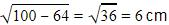QUESTION: 2

Solution:
QUESTION: 3

### In fig, O is the centre of the circle, CA is tangent at A and CB is tangent at B drawn to the circle. If ∠ACB = 75°, then ∠AOB =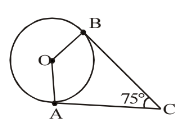Solution:
QUESTION: 4

In figure 10.75, PA and PB are the two tangents drawn to the circle. 0 is the centre of the circle. A and B are the points of contact of the tangents PA and PB with the circle. If ∠OPA = 35°, then∠ POB =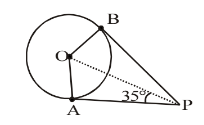Solution:
QUESTION: 5

In fig, O is the centre of the circle. PQ is tangent to the circle and secant PAB passes through the centre O. If PQ = 5 cm and PA = 1 cm, then the radius of the circle is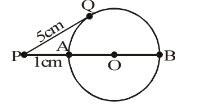Solution:
QUESTION: 6

A tangent PQ at a point P of a circle of radius 5 cm meets a line through the centre O at a point Q such that OQ = 12 cm. Length PQ is

Solution:
QUESTION: 7

From a point Q, the length of the tangent to a circle is 24 cm and the distance of Q from the centre is 25 cm. The radius of the circle is

Solution:
QUESTION: 8

The length of the tangent from a point A at a circle, of radius 3 cm, is 4 cm. The distance of A from the centre of the circle is

Solution:
QUESTION: 9

If tangents PA and PB from a point P to a circle with centre O are inclined to each other at an angle of 80° then ∠POA is equal to

Solution:
QUESTION: 10

If TP and TQ are two tangents to a circle with centre O so that ∠POQ = 110°, then, ∠PTQ is equal to

Solution:
QUESTION: 11

In the given figure, PT is tangent to the circle with centre O. If OT = 6 cm and OP = 10 cm then the length of tangent PT is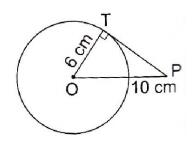Solution:

In right triangle PTO  By using Pythagoras theorem,PO2 = OT2 + TP

⇒ 102 = 62 + TP

⇒  TP = 100 - 36

⇒  TP = 64

⇒  TP  = 8

QUESTION: 12

Two circle touch each other externally at C and AB is a common tangent to the circles. Then, ∠ACB =

Solution:

Let P be a point on AB such that, PC is at right angles to the Line Joining the centers of the circles.

Note that, PC is a common tangent to both circles.

This is because tangent is perpendicular to radius at point of contact for any circle.

let ∠PAC= α and ∠PBC = β.

PA = PC [lengths of the tangents from an external point C]

In a triangle CAP, ∠PAC = ∠ACP = α

similarly PB = CP and ∠PCB = ∠CBP = β

now in the triangle ACB,

∠CAB + ∠CBA + ∠ACB = 180°   [sum of the interior angles in a triangle]

α + β + (α + β) = 180°   (Since ∠ACB = ∠ACP + ∠PCB = α + β.

2α + 2β = 180°

α + β = 90°

∴ ∠ACB = α + β = 90°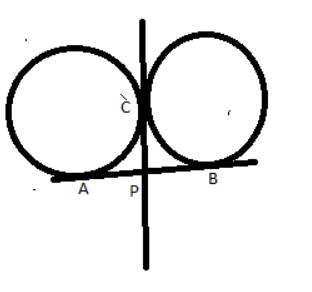QUESTION: 13

ABC is a right angled triangle, right angled at B such that BC = 6 cm and AB = 8 cm. A circle with centre O is inscribed in ΔABC. The radius of the circle is

Solution:
QUESTION: 14

PQ is a tangent drawn from a point P to a circle with centre O and QOR is a diameter of the circle such that ∠POR = 120°, then ∠OPQ is

Solution:
QUESTION: 15

If four sides of a quadrilateral ABCD are tangential to a circle, then

Solution:
QUESTION: 16

The length of the tangent drawn from a point 8 cm away from the centre of a circle of radius 6 cm is

Solution:

Let P be the external point and PA and PB be the tangents and OA and OB be the radii.
So OP is the hypotenuse=8cm
Applying Pythagoras theorem,
H= P+ B2
64 = AP+ 36
AP =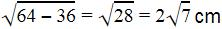QUESTION: 17

AB and CD are two common tangents to circles which touch each other at C. If D lies on AB such that CD = 4 cm, then AB is equal to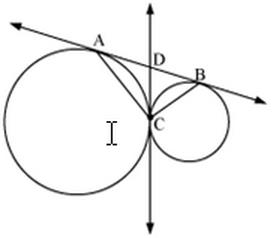Solution:

From the given figure, CD = 4 cm
CD=BD=4CD = BD = 4CD=BD=4 (tangents drawn from an exterior point are equal)

QUESTION: 18

In the adjoining figure, if AD, AE and BC are tangents to the circle at D, E and F respectively. Then,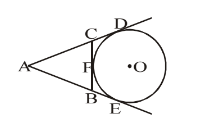Solution:
QUESTION: 19

If the diagonals of a cyclic quadrilateral are equal, then the quadrilateral is

Solution:

Let ABCD be a cyclic quadrilateral having diagonals BD and AC, intersecting each other at point O.

(Consider BD as a chord)

∠BCD = 180− 90 = 90
(Considering AC as a chord)

90+ ∠ABC = 180
∠ABC = 90

Each interior angle of a cyclic quadrilateral is of 90.Hence it is a rectangle.

QUESTION: 20

The quadrilateral formed by angle bisectors of a cyclic quadrilateral is a:

Solution:

To prove: PQRS is a cyclic quadrilateral.

Proof: In △ARB, we have

1/2∠A + 1/2∠B + ∠R = 180°   ....(i)   (Since, AR, BR are bisectors of ∠A and ∠B)

In △DPC, we have

1/2∠D + 1/2∠C +  ∠P = 180°  ....(ii)   (Since, DP,CP are bisectors of ∠D and ∠C respectively)

1/2∠A + 1/2∠B + ∠R + 1/2∠D + 1/2∠C + ∠P = 180° + 180°

∠P + ∠R = 360° - 1/2(∠A  + ∠B + ∠C  + ∠D)

∠P + ∠R = 360° - 1/2 x 360° = 360° - 180°

⇒ ∠P + ∠R = 180°

As the sum of a pair of opposite angles of quadrilateral PQRS is 180°. Therefore, quadrilateral PQRS is cyclic.

QUESTION: 21

In the given figure, AB is the diameter of the circle. Find the value of ∠ACD :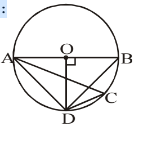Solution:
QUESTION: 22

Find the value of ∠DCE :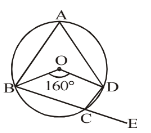Solution:
QUESTION: 23

In the given figure, PQ is the tangent of the circle. Line segment PR intersects the circle at N and R. PQ = 15 cm, PR = 25 cm, find PN: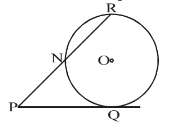Solution:

Given, PQ = 15 cm

PR = 25 cm

We know that, PQ² = PN × PR
15² = PN × 25
225 = PN × 25
PN = 9

QUESTION: 24

In the given figure, there are two circles with the centres O and O' touching each other internally at P. Tangents TQ and TP are drawn to the larger circle and tangents TP and TR are drawn to the smaller circle. Find TQ : TR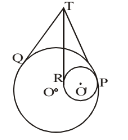Solution:
QUESTION: 25

In the given figure, PAQ is the tangent. BC is the diameter of the circle. m ∠BAQ = 60°, find m ∠ABC :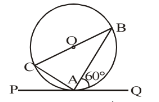Solution: